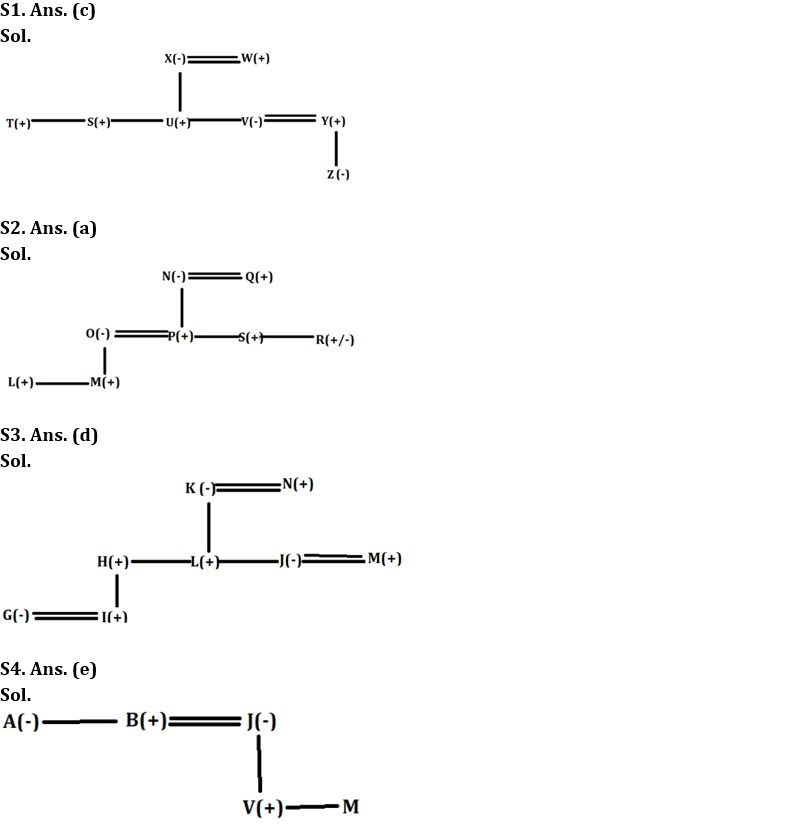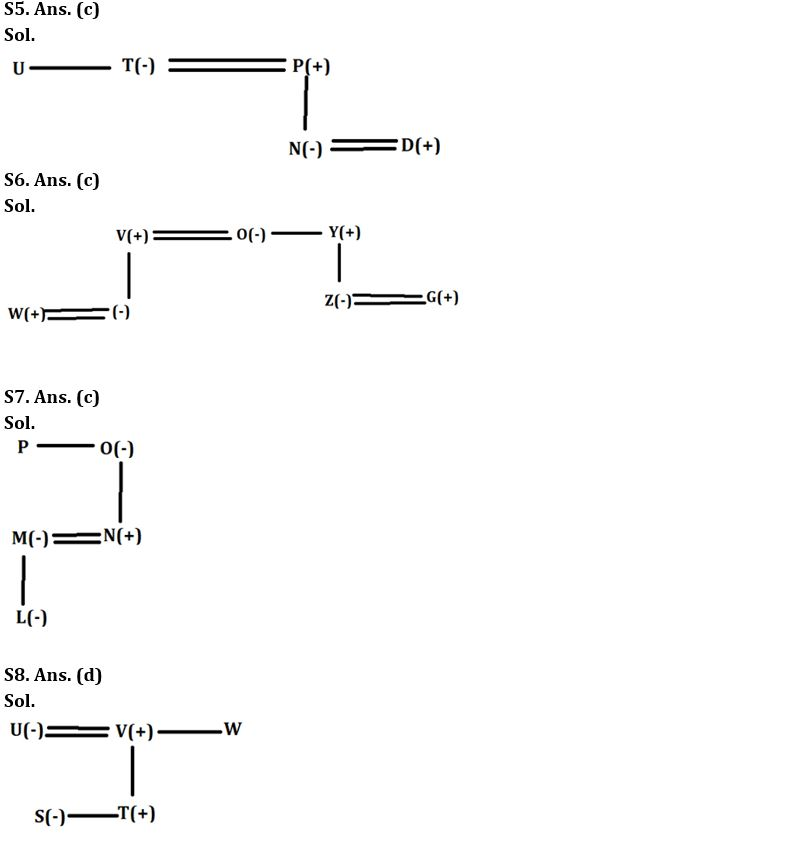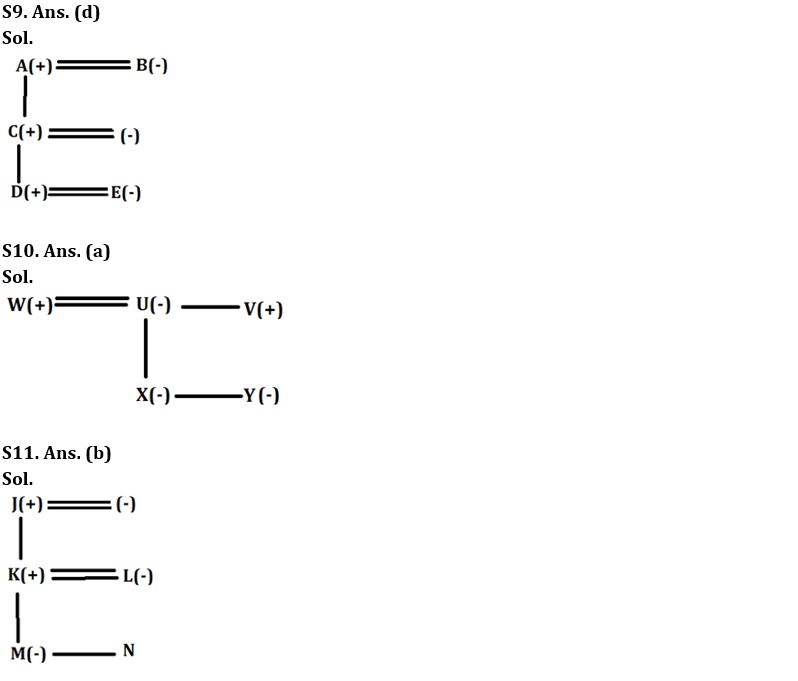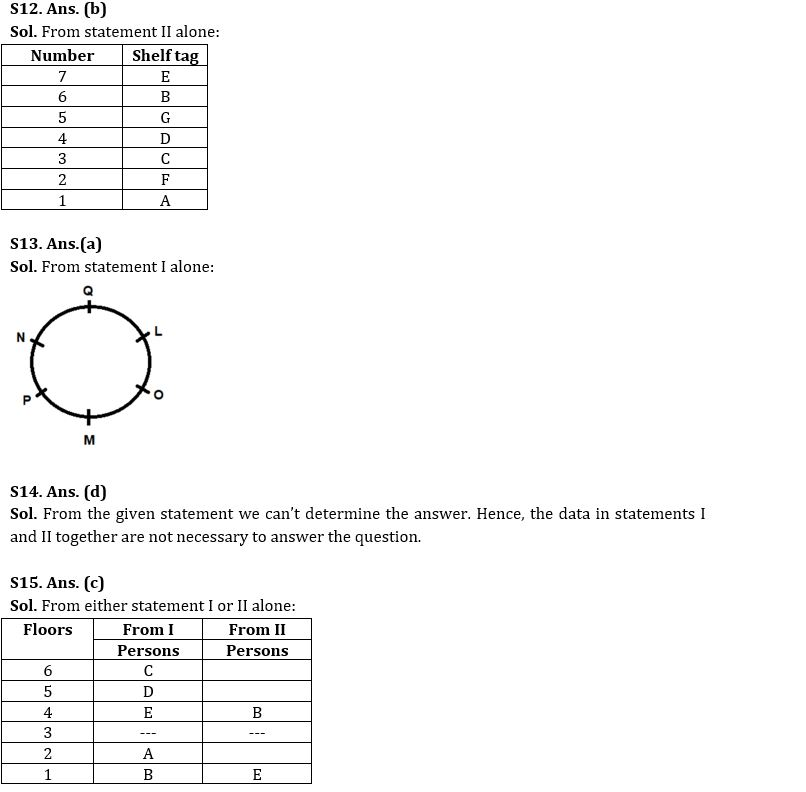Latest Banking jobs   »

# Reasoning Ability Quiz For SBI Clerk Mains 2022- 29th November

Directions (1-3): Answer the following questions based on the information given below.
If A#B means B is husband of A,
A@B means A is brother of B,
A ! B means A is son of B,
A\$B means A is parent of B

Q1. If ‘T@S@U!X #W\$V#Y\$ Z’ is true and there are at least three female members then how is Z related to T?
(a) Nephew
(b) Maternal Uncle
(c) Niece
(d) Daughter
(e) None of the above

Q2. If ‘L@M!O#P!N#Q\$S@R’ is true, then how is L related to mother of R?
(a) Grandson
(b) Grandfather
(c) Uncle
(d) Son
(e) Cannot be determined

Q3. If G#I!H@L!K#N\$J#M’ is true, then how is I related to wife of M?
(a) Niece
(b) Father
(c) Son
(d) Nephew
(e) None of the above

Directions (4-6): Answer the following questions based on the information given below.
In a coded language,
A+F means A is the husband of F
A= F means A is the brother of F
A*F means A is the sister of F
A / F means A is the mother of F
A% F means A is the father of F
A ( F means A is the wife of F
A÷ F means A is the son-in-law of F

Q4. If A*B+J/V=M, then how is M related to B?
(a) Daughter
(b) Brother
(c) Son
(d) Daughter-in-law
(e) Cannot be determined

Q5. If P% N(D ÷T*U, then how is P related to U?
(a) Father-in-law
(b) Nephew
(c) Brother-in-law
(d) Son
(e) Uncle

Q6. If ‘W÷V+O*Y%Z(G’ is true, then select the option which shows the pair of siblings in different gender?
(a) W-Z
(b) V-G
(c) Y-O
(d) Z-V
(e) G-Y

Directions (7-8): Answer the questions based on the information given below.
If, A % B means A is the wife of B;
A@B means A is the son of B;
A \$ B means A is the brother of B;
A & B means A is the daughter of B;
A*B means A is the sister of B.6

Q7. If L&M%N@O*P, then how is O related to L?
(a) Grandson
(b) Granddaughter
(c) Grandmother
(d) Grandfather
(e) Cannot be determined

Q8. If S*T@U%V\$W, how is S related to W?
(a) Uncle
(b) Aunt
(c) Nephew
(d) Niece
(e) Cannot be determined

If, X\$Y means ‘X is the husband of Y’
X@Y means ‘X is the mother of Y’
X&Y means ‘X is the sister of Y’
X%Y means ‘X is the brother-in-law of Y’
X#Y means ‘X is the father of Y’

Q9. How is A’s only daughter-in-law related to E in ‘A\$B@C#D\$E?
(a) Father
(b) Father-in-law
(c) Daughter
(d) Mother-in-law
(e) Cannot be determined

Q10. How is V related to X’s only sister in ‘U&V%W\$U@X&Y”?
(a) Uncle
(b) Aunt
(c) Father
(d) Niece
(e) Cannot be determined

Q11. How is L’s mother-in-law related to N in ‘J#K\$L@M&N’?
(a) Grandfather
(b) Grandmother
(c) Mother
(d) Father
(e) Cannot be determined

Direction (12-15): The question given below consists of two statements numbered I and II given below it. You have to decide whether the data provided in the statements are sufficient to answer the question. Read all the statements and give answer.

Q12. There are seven different shelves in a cupboard and each shelf is tagged as A, B, C, D, E, F and G. The shelf which is at the bottom, is numbered as 1, the shelf above it as 2 and so on. How many shelf/ shelves are below shelf B?
Statements:
I. A is 1st shelf. F is an even numbered shelf. G is 3 shelves above F. D is an even numbered shelf. C is immediately below D. E is above B.
II. E is 2 shelves above G which is a prime numbered shelf. E is not an even numbered shelf. A is 2 shelves below C which is a prime numbered shelf. B is above D which is above F.
(a) The data in statement I alone is sufficient to answer the question while the data in statement II alone is not sufficient to answer the question.
(b) The data in statement II alone is sufficient to answer the question while the data in statement I alone is not sufficient to answer the question.
(c) The data either in statement I alone or in statement II alone is sufficient to answer the question.
(d) The data given in statements I and II together are not sufficient to answer the question.
(e) The data in statements I and II together are necessary to answer the question.

Q13. Six people, L, M, N, O, P and Q, sit around a circular table such that the distance between two adjacent persons is the same. All the people face away from the centre. How many people sit between O and P when counted from the right of O?
Statements:
I. P sits opposite to L. O sits adjacent to L. Q sits immediate right of N. M does not sit opposite to N.
II. Q sits immediate left of L. M sits opposite to Q. Neither N nor P sits adjacent to O.
(a) The data in statement I alone is sufficient to answer the question while the data in statement II alone is not sufficient to answer the question.
(b) The data in statement II alone is sufficient to answer the question while the data in statement I alone is not sufficient to answer the question.
(c) The data either in statement I alone or in statement II alone is sufficient to answer the question.
(d) The data given in statements I and II together are not sufficient to answer the question.
(e) The data in statements I and II together are necessary to answer the question.

Q14. Certain number of persons are sitting in a linear row facing the north direction. How many persons sit to the right of O?
Statements:
I. Eight persons sit between G and T who sits immediate right of Z. Q sits third to the left of H who sits second from one of the ends of the row. Five persons sit between Z and Q.
II. Six persons sit between O and Z who sits exactly middle of the row. G sits fourth to the right of H who sits to the immediate left of N. Only two persons sit to the left of N.
(a) The data in statement I alone is sufficient to answer the question while the data in statement II alone is not sufficient to answer the question.
(b) The data in statement II alone is sufficient to answer the question while the data in statement I alone is not sufficient to answer the question.
(c) The data either in statement I alone or in statement II alone is sufficient to answer the question.
(d) The data given in statements I and II together are not sufficient to answer the question.
(e) The data in statements I and II together are necessary to answer the question.

Q15. Five friends A, B, C, D, and E live in a building having six floors numbered 1-6 from bottom to top i.e., ground floor is numbered as floor 1 and topmost floor is numbered as sixth floor. One of the floors is vacant. On which floor does E live? Neither B nor E lives on floor 5 or floor 6.
Statements:
I. D lives in between the floors on which C and E live. D lives immediately above E. Only one person lives in between B and E. Floor 3 is vacant. A lives on Floor 2.
II. Floor 3 is vacant. There are two floors between E’s floor and B’s floor. B lives above E.
(a) The data in statement I alone is sufficient to answer the question while the data in statement II alone is not sufficient to answer the question.
(b) The data in statement II alone is sufficient to answer the question while the data in statement I alone is not sufficient to answer the question.
(c) The data either in statement I alone or in statement II alone is sufficient to answer the question.
(d) The data given in statements I and II together are not sufficient to answer the question.
(e) The data in statements I and II together are necessary to answer the question.

Solutions#### Congratulations!Union Budget 2023-24: Free PDF